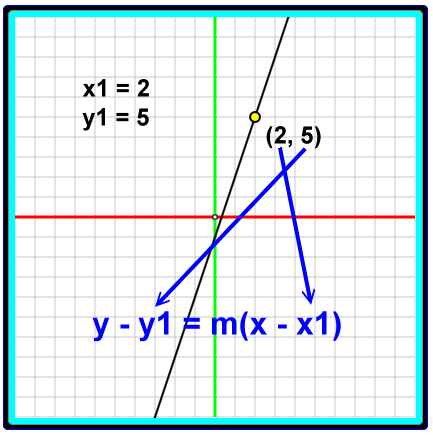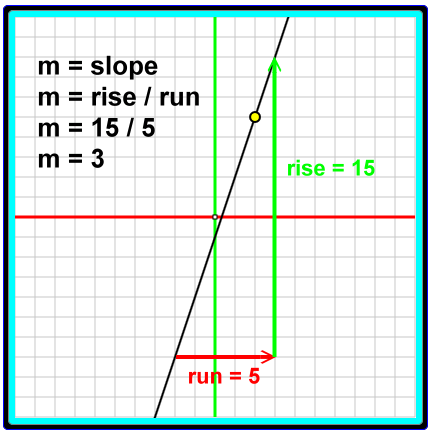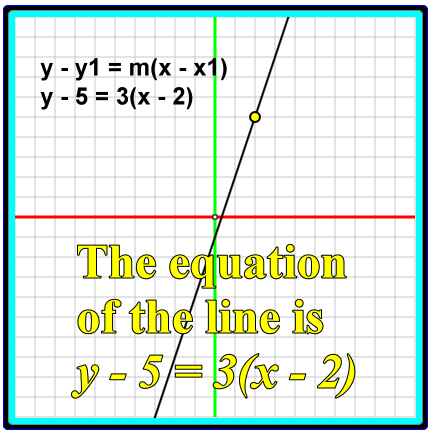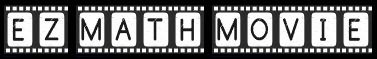# Linear Functions and Equations, Point-Slope Form

### y - y1 = m(x - x1)

The formula y - y1 = m(x - x1) is usually described as the 'point-slope form' for the equation of a line.

It is useful because if you know one point on a certain line and the slope of that certain line, then you can define the line with this type of formula and, thus, find all the other points on that certain line.

### Where is the point?

To use this equation you need to know one point on a certain line. The name of this known point is (x1, y1), and these x- and y-coordinate values are the numbers that appear, respectively, as x1 and y1 in the equation. Below the known point is shown by the yellow dot, and it has the coordinates (2, 5).### Where is the slope?

The slope of the line is the variable m. Below the slope of our line is calculated with a rise of 15 and a run of 5. The slope is equal to 3.### And the equation in point slope form is....### The following interactive application

The program below lets you change the known point coordinates and the slope for a line. You will be able to see how these changes effect the point-slope equation of the line, which is displayed over the graph, upper left.

This is an EZ Math Movie. Click the 'Show system' checkbox to expose the EZ Math Movie language. You do not have to understand EZ Math Movie to use this application.

0ptions

Go on input

Bounds:
Sorry, apparently this browser does not support HTML 5 canvas tag graphics.
Printer

Edit: Runtime          View: Starter Suggestion

To runtime:

Storage:

Editor width: Normal Wide

### Original page

The original page for this information contains a Java applet animation that resembles the application found here. If your browser runs Java, you may want to take a look at it. You can find it by clicking here.

Custom SearchThis page contains an EZ Math Movie application. EZ Math Movie is an animation programming language that helps you experiment with mathematics. Learn more about it at ezmathmovie.com

Name:
Domain:

Name
0

Name:
Name
0